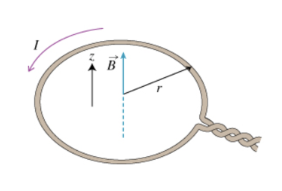# Problem: A piece of wire is bent to form a circle with radius r. It has a steady current I flowing through it in a counterclockwise direction as seen from the top (looking in the negative z direction). (Figure 1)Part AWhat is Bz(0), the z component of B⃗  at the center (i.e., x = y = z = 0) of the loop?Express your answer in terms of I, r, and constants like μ0 and π.

###### FREE Expert Solution

Magnetic field due to the element of current, idl at the center of the loop is expressed as:

$\overline{){\mathbf{d}}\stackrel{\mathbf{⇀}}{\mathbf{B}}{\mathbf{=}}\frac{{\mathbf{\mu }}_{\mathbf{0}}}{\mathbf{4}\mathbf{\pi }}{\mathbf{\left(}}\frac{\mathbf{i}\mathbf{d}\stackrel{\mathbf{⇀}}{\mathbf{l}}\mathbf{×}\stackrel{\mathbf{⇀}}{\mathbf{R}}}{{\mathbf{R}}^{\mathbf{3}}}{\mathbf{\right)}}}$

The vector R projects the element to center of the loop.

The magnitude of dB can be written as:

83% (408 ratings)###### Problem Details

A piece of wire is bent to form a circle with radius r. It has a steady current I flowing through it in a counterclockwise direction as seen from the top (looking in the negative direction). (Figure 1)Part A

What is Bz(0), the z component of B⃗  at the center (i.e., = 0) of the loop?

Express your answer in terms of Ir, and constants like μ0 and π.